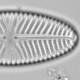## The missing rare taxa at Żabińskie

“rarity is the attribute of a vast number of species of all classes, in all countries.” Charles Darwin

In any census of any species-rich community, the rarest taxa are likely to be represented by a single individual. This can be visualised with rank abundance curves, shown here for Barro Colorado Island tree counts (jittered for clarity).In the BCI data, every sample contains many taxa represented by a single individual. Across all samples, 38% of taxon occurrences are of a single individual.

This is not the case for the Lake Żabińskie chironomid counts where 34 of 89 samples lack any taxa represented by a single (or a half) head.Not only do many samples lack singletons, one lacks any taxa occurring with fewer than five head capsules. There is a curious trend in the proportion of samples without singletons, with a higher prevelance of such samples in the second half of the record.These are the counts for the two most extreme cases.

```pos(fos_counts[minc == 5, ])
```
```##      Chironomini Microtendipes pedellus Cladotanytarsus mancus1
## 1967           7                      6                       6
##      Tanytarsus sp Tanytarsus lactesens Tanytarsus mendax
## 1967            11                    5                 6
```
```pos(fos_counts[minc == 4.5, ])
```
```##      Polypedilum nubeculosum Cladotanytarsus mancus1 Paratanytarsus
## 1970                     4.5                       8             12
## 1970           5          5                  4.5          4.5
```

How unlikely is it to have so many samples without singletons?

One way to test this is to fit a rank abundance model to the count data and then simulate assemblages from the model. There are a variety of rank abundance models that can be fitted with the `vegan` package. Here they are fitted to the first BCI sample.

```rf <- radfit(BCI[1, ])
plot(rf)
```Few singletons are expected with the pre-emption model, so I am going to apply this model to the chironomid counts and then simulate assemblages and test if they have singletons.

```fos_counts1 <- fos_counts
# promote half heads to full
fos_counts1[fos_counts1 %% 1 == 0.5] <- fos_counts1[fos_counts1 %% 1 == 0.5] + 0.5
#fit model and extract coefficients
alpha1 <- apply(fos_counts1, 1, function(r)  coef(rad.preempt(r)))

simulateMinAbun <- function(alpha, maxRank = 50, n = 10000, J = 30){
#maxRank is number of taxa considered, J is number of individuals, n is number of trials
rank <- 1:maxRank
abun <- J * alpha * (1 - alpha)^(rank - 1)
sims <- rmultinom(n = n, size = J, prob = abun)
table(apply(sims, 2, min0))/n
}
```
```high20 <- simulateMinAbun(max(alpha1), J = 20)
high30 <- simulateMinAbun(max(alpha1), J = 30)
high70 <- simulateMinAbun(max(alpha1), J = 70)

med30 <- simulateMinAbun(median(alpha1), J = 30)
phigh <- pbinom(q = sum(minc > 1), size = nrow(fos_counts), 1 - high30, lower.tail = FALSE)
pmed <- pbinom(q = sum(minc >  1), size = nrow(fos_counts), 1 - med30, lower.tail = FALSE)
```

For the sample with the largest coefficient for the pre-emption model (i.e. the steepest slope of the rank-abundance relationship), the probability of the count having at least one singleton is 0.91. This result is not sensitive to the number of heads counted (at least over the range reported for the chironomid data).

With the median pre-emption model coefficient, the probability of the count having at least one singleton is 0.9984.

Even in the most generous case, the probability of having the observed number of samples without singletons is 5 x 10-14. With the median case, the probability is 9 x 10-74.

This calculation does not account for the taxon inclusion rules (which were not adhered to) which would have removed taxa occurring in fewer than three samples. This would adjust the probability by several orders of magnitude. However, the calculation does not consider that in some samples neither singletons nor doubletons occur, which would adjust the probability by orders of magnitude in the other direction.

Whatever adjustments you want to make, the lack of rare taxa in the chironomid data is remarkable.

In the first version of the dataset, samples apparently missing singletons (identified by the minimum percent being above 2%) tended to have all their values as integer multiples of the minimum percent. This strongly suggested (as is now admitted) that the counts were not fifty as claimed, but much lower for some samples. Since then, the data have “evolved” and this integer multiple only holds for a few samples.

It is not obvious why there are so many samples without singletons. Perhaps the taxon inclusion rule (“3 percent in at least 3 samples”) was misapplied, smiting singletons in some levels only until the observed pattern emerged. At the calculated probabilities, almost anything is more likely than the data being correct.

It is trivial to infer that the currently archived data are definitely not the original data. The original data (all 76 taxa) needs to be archived.